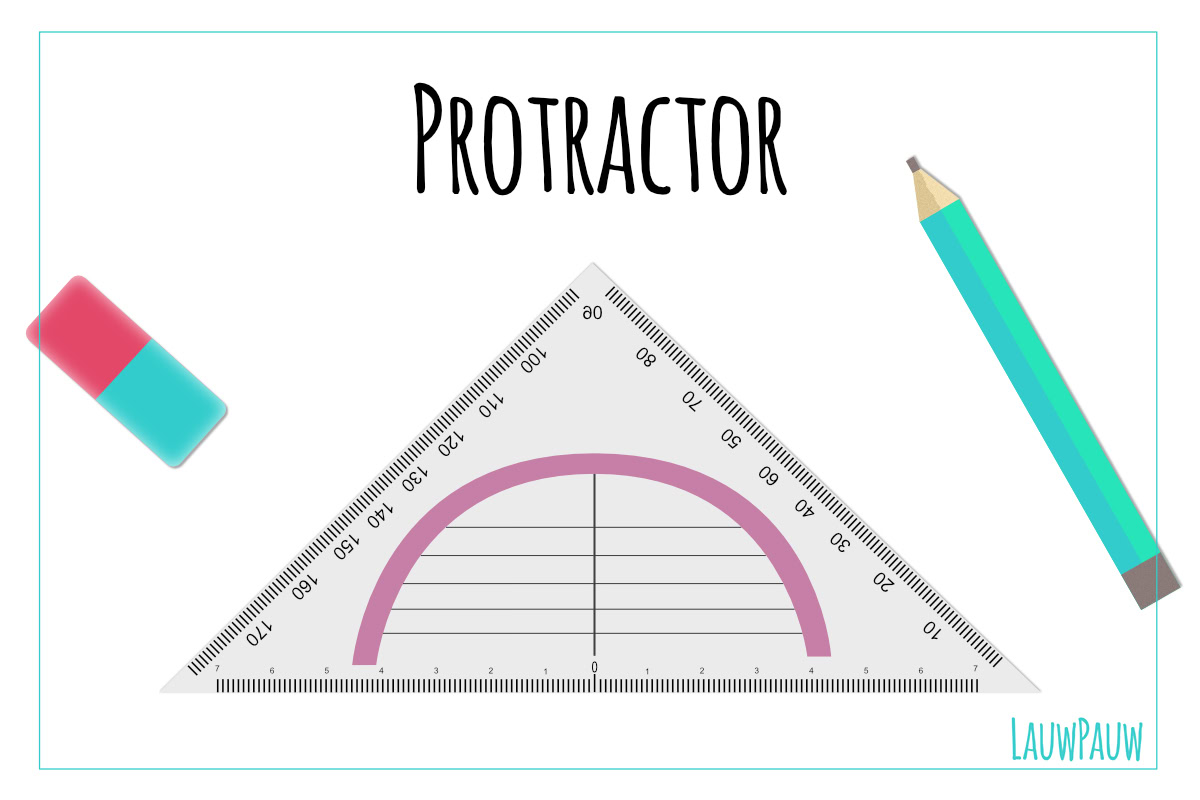# Protractor

How to draw lines under an angle or how can you make sure your lines are parallel to each other? There are a few important things. First, you’ll obviously need a protractor and a pencil mark which you can use to put light lines with, for example, HB or 2H.

This is a practical blog post so take some drawing paper so you immediately practice.

• drawing parallel lines.
• measuring angles, for example, to draw something in isometric or perspective.

## Oké, but how can you use this?

I’m going to explain and show you!

A triangle has a lot of numbers, and this can be used to draw a corner at a certain angle.

2. Put your protractor so that the 0 is located on the end of the line. (Fig. 1)
3. If you want to make an angle of 90 degrees (a right angle) put a dash in 90 (Fig. 2)
4. Then you put a line from the point where the 0 stood at the bar of the 90 (Fig. 3)
5. Done!

This can be used to draw many different angles, just look at the video, where I use my protractor to draw first 90-degree angles. Then I draw the sloping sides of my cube at an angle of 30 degrees. This means that all slanted sides exactly the same angle. First look at the video and then try yourself!

– The language in the video is in dutch, this will be updated soon –

## Parallel lines

You can also set up parallel lines with your protractor, which is easy to make lines equal to each run. For example, when drawing in isometric, all the lines run parallel. You can use the horizontal lines on your protractor for this.This site uses Akismet to reduce spam. Learn how your comment data is processed.